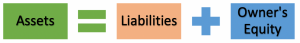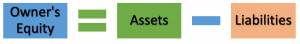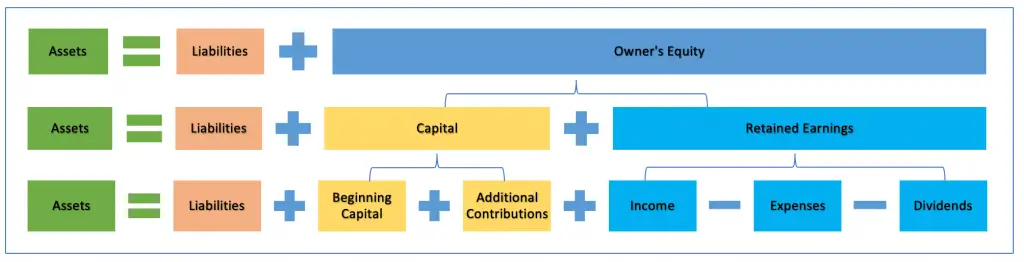# Accounting Equation

## Introduction

Accounting equation is the foundation of the double-entry in the accounting system which accounting transactions must follow. It is usually considered the most fundamental concept in the accounting system.

Accounting equation shows the relationship between balance sheet items including assets, liabilities and owner’s equity, in which total assets always equal to total liabilities plus total owner’s equity. Due to this, the accounting equation is also called the balance sheet equation sometimes.

## Accounting Equation Formula

The basic formula of accounting equation formula is assets equal to liabilities plus owner’s equity.Assets are what the company owns. They include cash on hand, cash at banks, investment, inventory, accounts receivable, prepaid, advance, fixed assets, etc.

Liabilities are what the company owes. They include accounts payable, tax payable, accrued expense, note payable, pension fund payable, etc.

Owner’s equity is the remaining of what the company has after deducting all liabilities from its total assets. Due to this, the owner’s equity is also known as net assets or net worth.

To easily understand and remember what the owner’s equity is, the accounting equation can be changed as:## Accounting Equation Example

For example, ABC Co. started the company on 02 January 2020 by injecting cash into the business of \$50,000. The \$30,000 came from its owner and \$20,000 came from the borrowing from the bank.

In this case, the company has total assets, its cash, \$50,000, liabilities of \$20,000 which is the borrowing from the bank and the owner’s equity of \$30,000. The accounting equation would look like below:

Assets = Liabilities + Owner’s Equity
\$50,000 = \$20,000 + \$30,000

If in one year, the company earned \$5,000 in cash from its business transactions. The figures in the accounting equation will change to:

Assets = Liabilities + Owner’s Equity
\$55,000 = \$20,000 + \$35,000

In this case, the total assets and owner’s equity increased \$5,000 while total liabilities are still the same.

In another case, if the company used \$5,000 to pay the loan principle to the bank. The figures in the accounting equation will be:

Assets = Liabilities + Owner’s Equity
\$50,000 = \$15,000 + \$35,000

In this case, the total assets are the same but the total liabilities decreased by \$5,000 while the owner’s equity increased by \$5,000

All in all, no matter the case, total assets will always equal total liabilities plus owner’s equity.

## Accounting Equation Concept

The basic concept of accounting equation is to express two main points in the accounting rule.

These two points include:

1. The total assets always equal the total liabilities plus owner’s equity
2. The relationship between balance sheet items

### Total assets always equal total liabilities plus owner’s equity

The concept here is that no matter what business transaction is, the accounting equation will always be balanced where total assets always equal total liabilities plus owner’s equity in the accounting.

In this case, there is no transaction that can make the equation not balanced. If there is, it would only mean one thing which is there is an error in accounting.

This concept helps the company to know where its assets (high level) come from and monitor its balance in the business. This is important as some companies may not be able to survive in the long term if their assets are mainly from liabilities while their equity is too small in comparison.

### Relationship between balance sheet items

Its concept is also to express the relationship of the balance sheet items which are assets, liabilities, and owner’s equity. As we have seen in the example above, the \$50,000 of cash which the owner injects into business becomes the assets of \$50,00.

And at the same time, the assets of \$50,000 have a direct relationship with liabilities of \$20,000 where the owner borrows from the bank; and the owner’s money of \$30,000 which becomes owner’s equity in the business.

## Expanded Accounting Equation

The concept of expanded accounting equation is that it shows further detail on where the owner’s equity comes from. In this case, the owner’s equity will be replaced with the elements that make it up.

These elements are basically capital and retained earnings; however, the expanded accounting equation is usually broken down further by replacing the retained earnings part with its elements. These include income, expenses, and dividends.

Additionally, the equation formula may also be broken down further on the capital part to detail the additional contributions of the capital. In this case, the capital will become the beginning capital and additional contributions.

All in all, we have expanded accounting equation formula which comes from basic accounting equation as below: